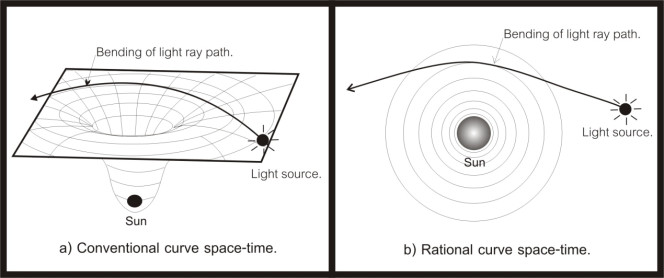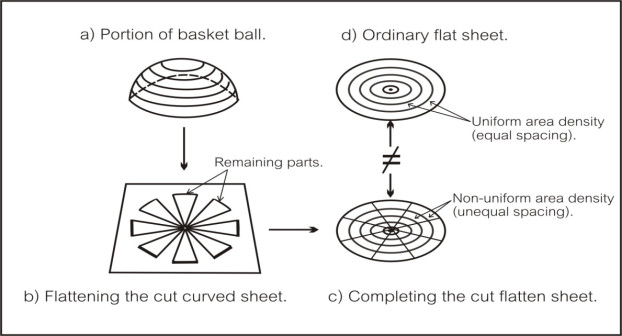# What is curved space, could we understand it?

Nimit Theeraleekul B. Eng. (Communication)
(1 July, 2011)

According to Einstein general theory of relativity (GTR), which said that gravity, is due to curved space caused by a nearby material mass. Anyway, there is no explanation why and how material mass create the surrounding curved space ! And because GTR is non-aether based theory in which vacuum space is an empty space, so it is very difficult to visualize how an empty curved space looks like . But if we could improve GTR to be aether based theory with vacuum medium space , then it is possible to understand the curved space via the non uniform of its density!

Here we will take a look for overall view; first we will summarize how Einstein had derived his conventional GTR as non aether-based theory. Next we will see why and how GTR has to change to aether-based theory, and finally we will see what we will gain from changing the using base concept of the theory. (Detail explanation could be found in “Completed Einstein general theory of relativity, CGTR” in www.vacuum-mechanics.com.)

1) Let us start with the idea that Einstein had extended GTR from STR, in which the principle of relativity (RP) is valid only in the realm of inertial system (i.e. constant moving frame relative to each other), to the principle of equivalence (EP) which cover system with noninertial reference frame (e.g. acceleration moving frame relative to each other).

Following the EP in GTR, the important of its meaning is that laws of physics are the same for observer in any system ! (While the RP in STR, its meaning is only that laws of physics are the same only for observer in inertial system .) Together with concept that an acceleration mass behave in the same way as the mass falling in gravitational field, then it guided Einstein to derive the famous “ gravitational field equation ” which I will call it as formula of the universe !

2) Next, according to the formula of the universe which state that the geometry of the universe's space-time is determined by the total mass and energy in the universe . This will make the nearby space around massive mass, such as the sun, curved in a way as show with rubber sheet in the diagram 1a. And when light ray (which normally move in a straight line) passing through this curved space, it will bend (follow the geodesic path) as shown. But the problem is that we cannot visualize how the space is curved via the idea of the rubber sheet!Figure 1 Light ray path through curved space-time

3) Now , if we accept that vacuum space is not an abstract empty space, instead it is a physical space of vacuum medium (something like aether), then we could improve the conventional non aether based GTR to be aether based theory. Armed with vacuum medium space, it is possible to understand the curved space via the non uniform of its density ! (Please see how to prove the existing of vacuum medium in the paper “ Einstein relativity vs. aether-based theory ”.)

To visualize how light ray path was bending while passing through vacuum medium space in the diagram 1b, it is easy done by using an analogy of the bending of light ray path in the earth atmosphere. Close to the earth surface, air density is denser than the far away air, so when light ray is passing from a smaller reflection index area (lower air's density) to a higher reflection index area (higher air's density), then it will bend down to the earth surface.

4) Up to now , we have see the new meaning of curved space via the non uniform density of vacuum medium space, but could we explain two-dimension curve surface such as a part of elastic basket ball surface in the new way? The answer is yes, we could explain it by using the diagram below.Figure 2 comparing a curve surface with a flat surface

In 2a), portion of curve surface of a basket ball was cut in order to flatten it as show in 2b. Then to make it as a complete flat sheet, we pull and glue all the cut sections together as in 2 c. Finally what we got is a completed flatten sheet with non-uniform area density, i.e. the sheet density will decrease where it is far away from the center. This is a new meaning of curve surface sheet – a non-uniform area density flat sheet surface ! Note that it is not the same thing as a normal flat surface sheet (with uniform density) as shown in 2d.

5) Now we will consider how material mass attract each other, such as the earth pulling an apple down, attract the moon or satellite in their orbits shown in diagram 3 below. Armed with the concept of vacuum medium space, it is easy to show that gravity is the mechanism of vacuum medium.

According to Newton or Einstein theories, gravity which shows in diagram 3a is a mysterious force without any mechanism. In contrast to the new concept of vacuum medium mechanism which is an understandable physical mechanism. Two part of the new mechanism involved; firstly gravity as its intrinsic property of material mass, secondly the gravity force is acting via vacuum medium – this is the mechanism of gravity!Figure 3 Attraction mechanism between two masses

(In CGTR we have make a reasonable assumption that vacuum medium is the fabric structure of our space, and to preserve the principle of conservation of energy (mass), vacuum medium must have gravity as its intrinsic property! Also we have proved that proton and electron (two elementary particles which form to be material mass) are condensed vacuum medium, and then it will act like tiny black holes.)

6 ) Up to now , we have seen that the modified (aether based) GTR theory can give us solution to the problem of gravity and curved space-time. Anyway there still have other mysterious unsolved problems such as dark energy and dark matter , which we also could explain it by using the new vacuum medium concept in the paper “Dark energy is in front of yours face”.

7) Another problem of GTR is about the speed of gravity wave , which said that it is the same as light speed. Anyway there is no explanation why it is so! Armed with the concept that light wave propagate via the oscillation in vacuum medium, then we can apply it to gravity wave as well. The reason is because any disturbance in vacuum medium will create a moving wave with constant speed c via the stress – strain relation in the medium with constant density!

8) One more problem is about the mechanism of what which was called as “ frame-dragging ” of space-time, which is the effect that a spinning object pulls space and time with it as it rotates. And recently, it was reported by Stanford and NASA researchers that the “Gravity Probe B” experiment test has confirmed the prediction of GTR.

Anyway, as mentioned early that vacuum space in GTR is an empty space, so it has the problem about the mechanism of curved space, here the situation is the same, and we do not know how empty space could be dragged along with the rotating object ? Instead if space is physical vacuum medium one, then this would be easy to visualize it work in the same way as the dragging of liquid around a rotating sphere!

By the way, it is interesting to note that there is a contradiction between the positive result of “ frame-dragging” got from general theory of relativity and the negative result of “aether drag” from special theory of relativity ! Armed with the aether based relativity, we know that the conventional interpretation of Michelson-Morley experiment (mentioned in “ Einstein relativity vs. aether-based theory “) is wrong, isn't it?